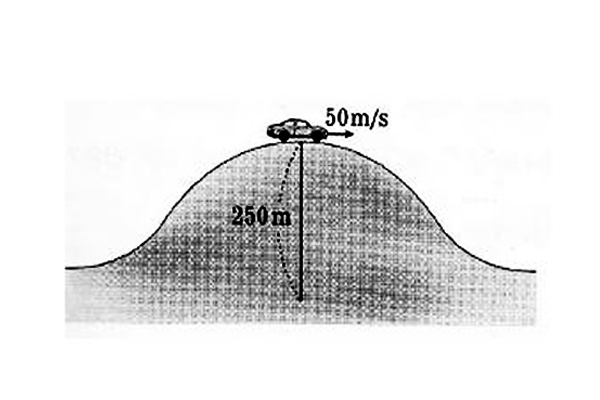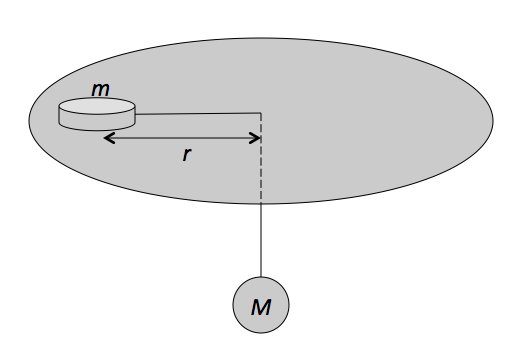Classical Mechanics

# Centripetal acceleration

A rock tied to a string is moving at a constant speed of $$8.0 \text{ m/s}$$ in a circle of radius $$7.0 \text{ m}.$$ Calculate the approximate magnitude of the centripetal acceleration of the rock.The above picture is a $$1000$$ kg car passing the top of a hill with radius of curvature $$250$$ m at a speed of $$50$$ m/s. Find the magnitude of normal force on the car (in N).

Gravitational acceleration is $$g= 10$$ m/s$$^{2}$$.A puck of mass $$m = 1.80 \text{ kg}$$ slides in a circle of radius $$r = 50.00 \text{ cm}$$ on a frictionless table while attached to a hanging ball of mass $$M = 3.50 \text{ kg}$$ by a cord through a hole in the table, as shown in the figure above. Approximately how fast must the puck move in order to keep the ball at rest?

The gravitational acceleration is $$g =10 \text{ m/s}^2.$$

A block is hung by a string from the roof of a van. The van drives along a straight, flat road at a constant speed of $$15 \sqrt{2} \text{ m/s},$$ and the block hangs vertically down. Then the van goes around an unbanked curve of radius $$45.0 \text{ m}$$ while maintaining the same speed, and the string tilts by an angle of $$\theta .$$ Find the value of $$\theta.$$

The gravitational acceleration is $$g= 10 \text{ m/s}^2.$$

A rock tied to a string is moving at a constant speed of $$1.8 \text{ m/s}$$ in a circle. If the rock's acceleration is $$3.6 \text{ m/s}^2,$$ what is the approximate radius of the circle it moves in?

×